Did you know you could have a single universal function that can replace all the functions in the world? All those sin(x), log(x), … whatever(x) can all be replaced by a single super function f(x). Don’t believe me? Just make those functions names – sin, log, … whatever to be another argument to that all-purpose function f, just like that: f(x, sin), f(x, log), … f(x, whatever). Now, we only must deal with a single function instead of many, and its second argument will define what transformation needs to be done with the first argument in order to arrive at this almighty function’s value.

## How many functions there are in SAS

Last time I counted there were more than 600 SAS functions, and that is excluding call routines and macro functions. But even that huge number grossly under-represents the actual number of functions available in SAS. That is because there are some functions that are built like the universal multi-purpose super function described above. For example, look at the following functions:

finance() function represents several dozen various financial functions;

finfo() function represents multiple functions returning various information items about files (file size, date created, date modified, access permission, etc.);

dinfo() function returns similar information items about directories;

attrn() function returns numeric attributes of a data set (number of observations, number of variables, etc.)

attrc() function returns character attributes of a data set (engine name, encoding name, character set, etc.)

Each of these functions represents not a single function, but a group of functions, and one of their arguments stipulates specific functionality (an information item or an attribute) that is being requested. You can think of this argument as a function modifier.

## %sysfunc SAS macro function

%sysfunc() is a super macro function that brings a wealth of SAS functions into SAS macro language. With very few exceptions, most SAS functions are available in SAS macro language thanks to the %sysfunc().

Moreover, we can build our own user-defined macro functions using SAS-supplied macro functions (such as %eval, %length, %quote, %scan, etc.), as well as hundreds of the SAS non-macro functions wrapped into the %sysfunc() super macro function.

## Building a super macro function to retrieve information about data sets

Armed with such a powerful arsenal, let’s build a multi-purpose macro function that taps into the data tables’ metadata and extracts various information items about those tables.

Let’s make this macro function return any of the following most frequently used values:

• Number of observations
• Number of variables
• Variables list (positional, separated by spaces)
• Variables list (positional, separated by commas)

Obviously, we can create much more of these information items and attributes, but here I am just showing how to do this so that you can create your own list depending on your needs.

In my earlier blog post, How to create and use SAS macro functions, we had already built a macro function for getting the number of observations; let’s expand on that.

Here is the SAS Macro code that handles extraction of all four specified metadata items:

```%macro dsinfo(dset,info); /* dset - data set name */ /* info - modifier (NOBS, NVARS, VARLIST, VARLISTC) */ %local dsid result infocaps i; %let infocaps = %upcase(&info); %let dsid = %sysfunc(open(&dset)); %if &dsid %then %do; %if &infocaps=NOBS %then %let result = %sysfunc(attrn(&dsid,nlobs)); %else %if &infocaps=NVARS %then %let result = %sysfunc(attrn(&dsid,nvars)); %else %if &infocaps=VARLIST %then %do i=1 %to %sysfunc(attrn(&dsid,nvars)); %let result = &result %sysfunc(varname(&dsid,&i)); %end; %else %if &infocaps=VARLISTC %then %do i=1 %to %sysfunc(attrn(&dsid,nvars)); %if &i eq 1 %then %let result = %sysfunc(varname(&dsid,&i)); %else %let result = &result,%sysfunc(varname(&dsid,&i)); %end; %let dsid = %sysfunc(close(&dsid)); %end; %else %put %sysfunc(sysmsg()); &result %mend dsinfo;```

The SAS log will show:

```%put NOBS=***%dsinfo(SASHELP.CARS,NOBS)***;
NOBS=***428***
%put NVARS=***%dsinfo(SASHELP.CARS,NVARS)***;
NVARS=***15***
%put VARLIST=***%dsinfo(SASHELP.CARS,VARLIST)***;
VARLIST=***Make Model Type Origin DriveTrain MSRP Invoice EngineSize Cylinders Horsepower MPG_City MPG_Highway Weight Wheelbase Length***
%put VARLISTC=***%dsinfo(SASHELP.CARS,VARLISTC)***;
VARLISTC=***Make,Model,Type,Origin,DriveTrain,MSRP,Invoice,EngineSize,Cylinders,Horsepower,MPG_City,MPG_Highway,Weight,Wheelbase,Length***```

## Macro function code highlights

We used the following statement to make our macro function case-insensitive regarding the info argument:

%let infocaps = %upcase(&info);

Then depending on the up-cased second argument of our macro function (modifier) we used the attrn(), varnum() and varname() functions within %sysfunc() to retrieve and construct our result macro variable.

We stick that result macro variable value, &result, right before the %mend statement so that the value is returned to the calling environment.

While info=VARLIST (space-separated variable list) is useful in DATA steps, info=VARLISTC (comma-separated variable list) is useful in PROC SQL.

## Usage example

Having this %dsinfo macro function at hands, we can use it in multiple programming scenarios. For example:

```/* ending SAS session if no observations to process */ %if %dsinfo(SASHELP.CARS,NOBS)=0 %then %do; endsas; %end;   /* further processing */ data MYNEWDATA (keep=%dsinfo(SASHELP.CARS,VARLIST)); retain %dsinfo(SASHELP.CARS,VARLIST); set SASHELP.CARS; if _n_=1 then put %dsinfo(SASHELP.CARS,VARLIST); /* ... */ run;```

Here we first check if there is at least one observation in a data set. If not (0 observations) then we stop the SAS session and don’t do any further processing. Otherwise, when there are some observations to process, we continue.

If SAS code needs multiple calls to the same macro function with the same argument, we can shorten the code by first assigning that macro function’s result to a macro variable and then reference that macro variable instead of repeating macro function invocation. Here is an example:

```/* further processing */ %let vlist = %dsinfo(SASHELP.CARS,VARLIST); data MYNEWDATA (keep=&vlist); retain &vlist; set SASHELP.CARS; if _n_=1 then put &vlist; /* ... */ run;```

Do you see the benefits of these multi-purpose SAS macro functions? Can you suggest other scenarios of their usage? Please share your thoughts in the comments section below.If you have been learning about machine learning or mathematical statistics, you might have heard about the Kullback–Leibler divergence. The Kullback–Leibler divergence is a measure of dissimilarity between two probability distributions. It measures how much one distribution differs from a reference distribution. This article explains the Kullback–Leibler divergence and shows how to compute it for discrete probability distributions.

Recall that there are many statistical methods that indicate how much two distributions differ. For example, a maximum likelihood estimate involves finding parameters for a reference distribution that is similar to the data. Statistics such as the Kolmogorov-Smirnov statistic are used in goodness-of-fit tests to compare a data distribution to a reference distribution.

### What is the Kullback–Leibler divergence?

Let f and g be probability mass functions that have the same domain. The Kullback–Leibler (K-L) divergence is the sum
KL(f, g) = Σx f(x) log( f(x)/g(x) )
where the sum is over the set of x values for which f(x) > 0. (The set {x | f(x) > 0} is called the support of f.) The K-L divergence measures the similarity between the distribution defined by g and the reference distribution defined by f.

For this sum to be well defined, the distribution g must be strictly positive on the support of f. That is, the Kullback–Leibler divergence is defined only when g(x) > 0 for all x in the support of f.

Some researchers prefer the argument to the log function to have f(x) in the denominator. Flipping the ratio introduces a negative sign, so an equivalent formula is
KL(f, g) = –Σx f(x) log( g(x)/f(x) )

Notice that if the two density functions (f and g) are the same, then the logarithm of the ratio is 0. Therefore, the K-L divergence is zero when the two distributions are equal. The K-L divergence is positive if the distributions are different.

The Kullback–Leibler divergence was developed as a tool for information theory, but it is frequently used in machine learning. The divergence has several interpretations. In information theory, it measures the information loss when f is approximated by g. In statistics and machine learning, f is often the observed distribution and g is a model.

### An example of Kullback–Leibler divergence

As an example, suppose you roll a six-sided die 100 times and record the proportion of 1s, 2s, 3s, etc. You might want to compare this empirical distribution to the uniform distribution, which is the distribution of a fair die for which the probability of each face appearing is 1/6. The following SAS/IML statements compute the Kullback–Leibler (K-L) divergence between the empirical density and the uniform density:

```proc iml; /* K-L divergence is defined for positive discrete densities */ /* Face: 1 2 3 4 5 6 */ f = {20, 14, 19, 19, 12, 16} / 100; /* empirical density; 100 rolls of die */ g = { 1, 1, 1, 1, 1, 1} / 6; /* uniform density */ KL_fg = -sum( f#log(g/f) ); /* K-L divergence using natural log */ print KL_fg;```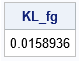The K-L divergence is very small, which indicates that the two distributions are similar.

Although this example compares an empirical distribution to a theoretical distribution, you need to be aware of the limitations of the K-L divergence. The K-L divergence compares two distributions and assumes that the density functions are exact. The K-L divergence does not account for the size of the sample in the previous example. The computation is the same regardless of whether the first density is based on 100 rolls or a million rolls. Thus, the K-L divergence is not a replacement for traditional statistical goodness-of-fit tests.

### The Kullback–Leibler is not symmetric

Let's compare a different distribution to the uniform distribution. Let h(x)=9/30 if x=1,2,3 and let h(x)=1/30 if x=4,5,6. The following statements compute the K-L divergence between h and g and between g and h. In the first computation, the step distribution (h) is the reference distribution. In the second computation, the uniform distribution is the reference distribution.

```h = { 9, 9, 9, 1, 1, 1} /30; /* step density */ g = { 1, 1, 1, 1, 1, 1} / 6; /* uniform density */ KL_hg = -sum( h#log(g/h) ); /* h is reference distribution */ print KL_hg;   /* show that K-L div is not symmetric */ KL_gh = -sum( g#log(h/g) ); /* g is reference distribution */ print KL_gh;```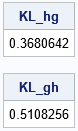First, notice that the numbers are larger than for the example in the previous section. The f density function is approximately constant, whereas h is not. So the distribution for f is more similar to a uniform distribution than the step distribution is. Second, notice that the K-L divergence is not symmetric. In the first computation (KL_hg), the reference distribution is h, which means that the log terms are weighted by the values of h. The weights from h give a lot of weight to the first three categories (1,2,3) and very little weight to the last three categories (4,5,6). In contrast, g is the reference distribution for the second computation (KL_gh). Because g is the uniform density, the log terms are weighted equally in the second computation.

The fact that the summation is over the support of f means that you can compute the K-L divergence between an empirical distribution (which always has finite support) and a model that has infinite support. However, you cannot use just any distribution for g. Mathematically, f must be absolutely continuous with respect to g. (Another expression is that f is dominated by g.) This means that for every value of x such that f(x)>0, it is also true that g(x)>0.

### A SAS function for the Kullback–Leibler divergence

It is convenient to write a function, KLDiv, that computes the Kullback–Leibler divergence for vectors that give the density for two discrete densities. The call KLDiv(f, g) should compute the weighted sum of log( g(x)/f(x) ), where x ranges over elements of the support of f. By default, the function verifies that g > 0 on the support of f and returns a missing value if it isn't. The following SAS/IML function implements the Kullback–Leibler divergence.

```/* The Kullback–Leibler divergence between two discrete densities f and g. The f distribution is the reference distribution, which means that the sum is probability-weighted by f. If f(x0)>0 at some x0, the model must allow it. You cannot have g(x0)=0. */ start KLDiv(f, g, validate=1); if validate then do; /* if g might be zero, validate */ idx = loc(g<=0); /* find locations where g = 0 */ if ncol(idx) > 0 then do; if any(f[idx]>0) then do; /* g is not a good model for f */ *print "ERROR: g(x)=0 when f(x)>0"; return( . ); end; end; end; if any(f<=0) then do; /* f = 0 somewhere */ idx = loc(f>0); /* support of f */ fp = f[idx]; /* restrict f and g to support of f */ return -sum( fp#log(g[idx]/fp) ); /* sum over support of f */ end; /* else, f > 0 everywhere */ return -sum( f#log(g/f) ); /* K-L divergence using natural logarithm */ finish;   /* test the KLDiv function */ f = {0, 0.10, 0.40, 0.40, 0.1}; g = {0, 0.60, 0.25, 0.15, 0}; KL_pq = KLDiv(f, g); /* g is not a valid model for f; K-L div not defined */ KL_qp = KLDiv(g, f); /* f is valid model for g. Sum is over support of g */ print KL_pq KL_qp;```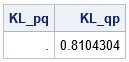The first call returns a missing value because the sum over the support of f encounters the invalid expression log(0) as the fifth term of the sum. The density g cannot be a model for f because g(5)=0 (no 5s are permitted) whereas f(5)>0 (5s were observed). The second call returns a positive value because the sum over the support of g is valid. In this case, f says that 5s are permitted, but g says that no 5s were observed.

### Summary

This article explains the Kullback–Leibler divergence for discrete distributions. A simple example shows that the K-L divergence is not symmetric. This is explained by understanding that the K-L divergence involves a probability-weighted sum where the weights come from the first argument (the reference distribution). Lastly, the article gives an example of implementing the Kullback–Leibler divergence in a matrix-vector language such as SAS/IML. This article focused on discrete distributions. The next article shows how the K-L divergence changes as a function of the parameters in a model.

### References

The post The Kullback–Leibler divergence between discrete probability distributions appeared first on The DO Loop.Fig.1.雷达图解析 (出自The Data Visualisation Catalogue)

Fig.2. 电脑系统资源使用情况示意图

1，由于不是规则的坐标图，即极坐标图，所以数据得转换，从XY坐标图转换到极坐标图，因此数据要完成角度弧度的转换；

2，只有一个极坐标轴了，坐标轴变成了辐条，可以用sgplot vector来完成；坐标轴上的刻度及参考线可以用polygon画多边形或ellipseparm画圆完成；数据点用scatter完成；数据点之间的连线用polygon多边形来完成；

• data ex;
• do situation = 1 to 0 by –1;
•  input cpu memory disk gpu network;output;
•  end;
• cards;
• 20 20 90 10 10
• 50 50 50 50 50
• ;
• run;
• proc sort data=ex out=ex;by situation;run;
• proc transpose data=ex out= ex2 name=config prefix= use ;
• by situation;
• run;

Fig.3. 期望的实际数据形式

• data ex3;
•      set ex2;
•        deg   =(90360/5*(_N_-1)) ; /*  5由变量个数来决定   */
•        rangle =  –360/5*(_N_-1)  ;/*  5由变量个数来决定   */
•        rdx = use1*cos(angle) ;
•        rdy = use1*sin(angle) ;
•        rx = 100*cos(angle); ry=100*sin(angle);/*100由实际情况定 */
•        config=upcase(config);
•        if situation then situ_=”实际情况” ;else situ_=”理性情况”;
• run;

Fig.4. 转换的实际数据和构图数据形式

• ods listing image_dpi=600 gpath=”d:”   ;
• ods graphics on  /  imagename=”Figure_”  HEIGHT= 7 cm WIDTH= 7 cm border=no ;
• footnote;
• title;
• proc sgplot data=ex3  ASPECT=1  noborder  SUBPIXEL;
• STYLEATTRS DATACONTRASTCOLORS=(blue red);
•     polygon x=rdx y=rdy id=situation/ group=situ_ outline LINEATTRS=(pattern=solid color=blue THICKNESS=2) fill fillattrs=(transparency=0.) name=”sxlion”;
•       vector  x=rx y=ry / xOrigin=0 yOrigin=0  NOARROWHEADS LINEATTRS=(pattern=solid color=black THICKNESS=1.5) ;
•       text x=rx y=ry text=config / ROTATE=rangle position=top textattrs= (Color=darkblue Family=”Helvetica” Size=10 Weight=Bold);
•       ellipseparm SEMIMAJOR=50 SEMIMINOR=50;/*中刻度线，自定*/
•       ellipseparm SEMIMAJOR=99 SEMIMINOR=99;/*外刻度线，自定*/
•       scatter x=rdx y=rdy /markerattrs=(symbol=circlefilled color=red Size=6);
• keylegend  “sxlion” / noborder;
• xaxis DISPLAY=none;
• yaxis DISPLAY=none;
• run;
• ods graphics off;

SAS绘图sg系列语句可以模块化，多个功能叠加在一起就可以组成一个雷达图了。当然，既然是组合，只要兼容的sg系列语句都可以叠加在一起，并且按语句出现的先后顺序叠加起来，语句的前后顺序可根据需要进行安排。

This month we celebrated International Nurses Day at a time when nurses and other health care professionals have never been so needed. This important day also fell on what would have been Florence Nightingale’s 200th birthday. Most people know Florence Nightingale as the famous nurse who saved hundreds of lives during the [...]

Channeling Nightingale in the time of COVID-19 was published on SAS Voices by Gale Adcock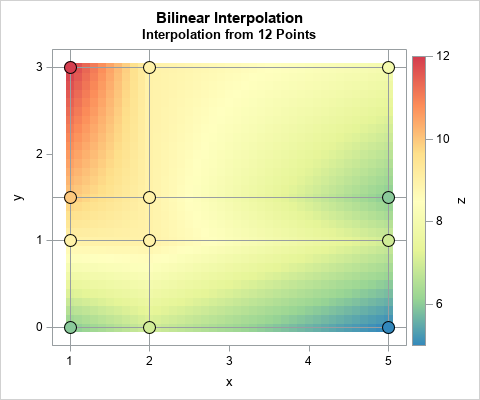This article shows how to perform two-dimensional bilinear interpolation in SAS by using a SAS/IML function. It is assumed that you have observed the values of a response variable on a regular grid of locations.

A previous article showed how to interpolate inside one rectangular cell. When you have a grid of cells and a point (x, y) at which to interpolate, the first step is to efficiently locate which cell contains (x, y). You can then use the values at the corners of the cell to interpolate at (x, y), as shown in the previous article. For example, the adjacent graph shows the bilinear interpolation function that is defined by 12 points on a 4 x 3 grid.

### Bilinear interpolation assumptions

For two-dimensional linear interpolation of points, the following sets of numbers are the inputs for bilinear interpolation:

1. Grid locations: Let x1 < x2 < ... < xp be the horizontal coordinates of a regular grid. Let y1 < y2 < ... < yn be the vertical coordinates.
2. Response Values: Let Z be an n x p matrix of response values on the grid. The (j, i)th value of Z is the response value at the location (xi, yj). Notice that the columns of Z correspond to the horizontal positions for the X values and the rows of Z correspond to the vertical positions for the Y values. (This might seem "backwards" to you.) The response values data should not contain any missing values. The X, Y, and Z values (called the "fitting data") define the bilinear interpolation function on the rectangle [x1, xp] x [y1, yn].
3. Values to score: Let (s1, t1), (s2, t2), ..., (sk, tk) be a set of k new (x, y) values at which you want to interpolate. All values must be within the range of the data: x1 ≤ si ≤ xp and y1 ≤ si ≤ yn for all (i,j) pairs. These values are called the "scoring data" or the "query data."

The goal of interpolation is to estimate the response at each location (si, tj) based on the values at the corner of the cell that contains the location. I have written a SAS/IML function called BilinearInterp, which you can freely download and use. The following statements indicate how to define and store the bilinearInterp function:

```proc iml; /* xGrd : vector of Nx points along x axis yGrd : vector of Ny points along y axis z : Ny x Nx matrix. The value Z[i,j] is the value at xGrd[j] and yGrd[i] t : k x 2 matrix of points at which to interpolate The function returns a k x 1 vector, which is the bilinear interpolation at each row of t. */ start bilinearInterp(xgrd, ygrd, z, t); /* download function from https://github.com/sascommunities/the-do-loop-blog/blob/master/interpolation/bilinearInterp.sas */ finish; store module=bilinearInterp; /* store the module so it can be loaded later */ QUIT;```

### The structure of the fitting data

The fitting data for bilinear interpolation consists of the grid of points (Z) and the X and Y locations of each grid point. The X and Y values do not need to be evenly spaced, but they can be.

Often the fitting data are stored in "long form" as triplets of (X, Y, Z) values. If so, the first step is to read the triplets and convert them into a matrix of Z values where the columns are associated with the X values and the rows are associated with the Y values. For example, the following example data set specifies a response variable (Z) on a 4 x 3 grid. The values of the grid in the Y direction are {0, 1, 1.5, 3} and the values in the X direction are {1, 2, 5}.

As explained in the previous article, the data must be sorted by Y and then by X. The following statements read the data into a SAS/IML matrix and extract the grid points in the X and Y direction:

```data Have; input x y z; datalines; 1 0 6 2 0 7 5 0 5 1 1 9 2 1 9 5 1 7 1 1.5 10 2 1.5 9 5 1.5 6 1 3 12 2 3 9 5 3 8 ;   /* If the data are not already sorted, sort by Y and X */ proc sort data=Have; by Y X; run;   proc iml; use Have; read all var {x y z}; close; xGrd = unique(x); /* find grid points */ yGrd = unique(y); z = shape(z, ncol(yGrd), ncol(xGrd)); /* data must be sorted by y, then x */ print z[r=(char(yGrd)) c=(char(xGrd))];```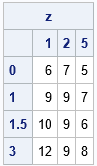Again, note that the columns of Z represent the X values and the rows represent the Y values. The xGrd and yGrd vectors contain the grid points along the horizontal and vertical dimensions of the grid, respectively.

### The structure of the scoring data

The bilinearInterp function assumes that the points at which to interpolate are stored in a k x 2 matrix. Each row is an (x,y) location at which to interpolate from the fitting data. If an (x,y) location is outside of the fitting data, then the bilinearInterp function returns a missing value. The scoring locations do not need to be sorted.

The following example specifies six valid points and two invalid interpolation points:

```t = {0 0, /* not in data range */ 1 1, 1 2, 2.5 2, 4 0.5, 4 2, 5 3, 6 3}; /* not in data range */   /* LOAD the previously stored function */ load module=bilinearInterp; F = bilinearInterp(xGrd, yGrd, z, t); print t[c={'x' 'y'}] F[format=Best4.];```The grid is defined on the rectangle [1,5] x [0,3]. The first and last rows of t are outside of the rectangle, so the interpolation returns missing values at those locations. The locations (1,1) and (5,3) are grid locations (corners of a cell), and the interpolation returns the correct response values. The other locations are in one of the grid cells. The interpolation is found by scaling the appropriate rectangle onto the unit square and applying the bilinear interpolation formula, as shown in the previous article.

### Visualizing the bilinear interpolation function

You can use the ExpandGrid function to generate a fine grid of locations at which to interpolate. You can visualize the bilinear interpolation function by using a heat map or by using a surface plot.

The heat map visualization is shown at the top of this article. The colors indicate the interpolated values of the response variable. The markers indicate the observed values of the response variable. The reference lines show the grid that is defined by the fitting data.

The following graph visualizes the interpolation function by using a surface plot. The function is piecewise quadratic. Within each cell, it is linear on lines of constant X and constant Y. The "ridge lines" on the surface correspond to the locations where two adjacent grid cells meet.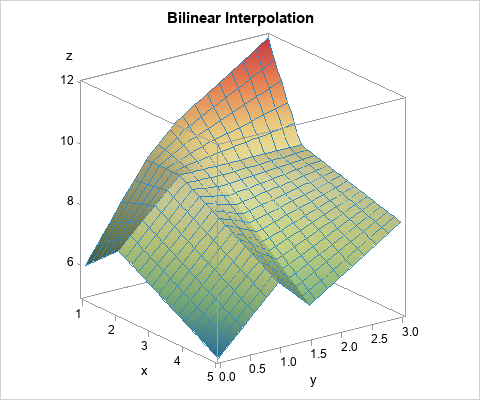### Summary

In summary, you can perform bilinear interpolation in SAS by using a SAS/IML function. The function uses fitting data (X, Y, and Z locations on a grid) to interpolate. Inside each cell, the interpolation is quadratic and is linear on lines of constant X and constant Y. For each location point, the interpolation function first determines what cell the point is in. It then uses the corners of the cell to interpolate at the point. You can download the SAS program that performs bilinear interpolation and generates the tables and graphs in this article.

The post Bilinear interpolation in SAS appeared first on The DO Loop.SAS partners with Appalachian State University on the World Bee Count initiative to support bee health.

Count and save the bees with analytics was published on SAS Voices by Lee Ellen Harmer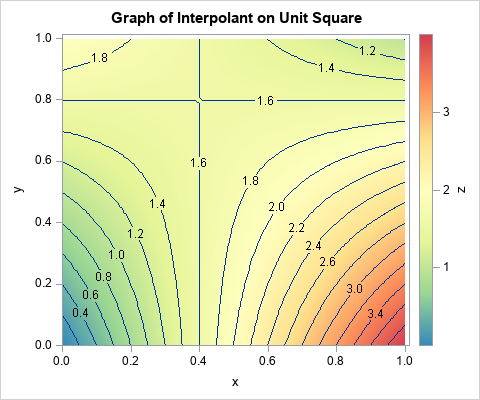I've previously written about linear interpolation in one dimension. Bilinear interpolation is a method for two-dimensional interpolation on a rectangle. If the value of a function is known at the four corners of a rectangle, an interpolation scheme gives you a way to estimate the function at any point in the rectangle's interior. Bilinear interpolation is a weighted average of the values at the four corners of the rectangle. For an (x,y) position inside the rectangle, the weights are determined by the distance between the point and the corners. Corners that are closer to the point get more weight. The generic result is a saddle-shaped quadratic function, as shown in the contour plot to the right.

The Wikipedia article on bilinear interpolation provides a lot of formulas, but the article is needlessly complicated. The only important formula is how to interpolate on the unit square [0,1] x [0,1]. To interpolate on any other rectangle, simply map your rectangle onto the unit square and do the interpolation there. This trick works for bilinear interpolation because the weighted average depends only on the relative position of a point and the corners of the rectangle. Given a rectangle with lower-left corner (x0, y0) and upper right corner (x1, y1), you can map it into the unit square by using the transformation (x, y) → (u, v) where u = (x-x0)/(x1-x0) and v = (y-y0)/(y1-y0).

### Bilinear interpolation on the unit square

Suppose that you want to interpolate on the unit square. Assume you know the values of a continuous function at the corners of the square:

• z00 is the function value at (0,0), the lower left corner
• z10 is the function value at (1,0), the lower right corner
• z01 is the function value at (0,1), the upper left corner
• z11 is the function value at (1,1), the upper right corner

If (x,y) is any point inside the unit square, the interpolation at that point is the following weighted average of the values at the four corners:
F(x,y) = z00*(1-x)*(1-y) + z10*x*(1-y) + z01*(1-x)*y + z11*x*y

Notice that the interpolant is linear with respect to the values of the corners of the square. It is quadratic with respect to the location of the interpolation point. The interpolant is linear along every horizontal line (lines of constant y) and along every vertical line (lines of constant x).

### Implement bilinear interpolation on the unit square in SAS

Suppose that the function values at the corners of a unit square are z00 = 0, z10 = 4, z01 = 2, and z11 = 1. For these values, the bilinear interpolant on the unit square is shown at the top of this article. Notice that the value of the interpolant at the corners agrees with the data values. Notice also that the interpolant is linear along each edge of the square. It is not easy to see that the interpolant is linear along each horizontal and vertical line, but that is true.

In SAS, you can use the SAS/IML matrix language to define a function that performs bilinear interpolation on the unit square. The function takes two arguments:

• z is a four-element that specifies the values of the data at the corners of the square. The values are specified in the following order: lower left, lower right, upper left, and upper right. This is the "fitting data."
• t is a k x 2 matrix, where each row specifies a point at which to interpolate. This is the "scoring data."

In a matrix language such as SAS/IML, the function can be written in vectorized form without using any loops:

```proc iml; start bilinearInterpSquare(z, t); z00 = z; z10 = z; z01 = z; z11 = z; x = t[,1]; y = t[,2]; cx = 1-x; /* cx = complement of x */ return( (z00*cx + z10*x)#(1-y) + (z01*cx + z11*x)#y ); finish;   /* specify the fitting data */ z = {0 4, /* bottom: values at (0,0) and (1,0) */ 2 1}; /* top: values at (0,1) and (1,1) */ print z[c={'x=0' 'x=1'} r={'y=0' 'y=1'}];   t = {0.5 0, /* specify the scoring data */ 0 0.25, 1 0.66666666, 0.5 0.75, 0.75 0.5 }; F = bilinearInterpSquare(z, t); /* test the bilinear interpolation function */ print t[c={'x' 'y'} format=FRACT.] F;```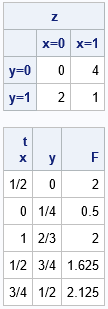For points on the edge of the square, you can check a few values in your head:

• The point (1/2, 0) is halfway between the corners with values 0 and 4. Therefore the interpolation gives 2.
• The point (0, 1/4) is a quarter of the way between the corners with values 0 and 2. Therefore the interpolation gives 0.5.
• The point (1, 2/3) is two thirds of the way between the corners with values 4 and 1. Therefore the interpolation gives 2.

The other points are in the interior of the square. The interpolant at those points is a weighted average of the corner values.

### Visualize the interpolant function

Let's use the bilinearInterpSquare function to evaluate a grid of points. You can use the ExpandGrid function in SAS/IML to generate a grid of points. When we look at the values of the interpolant on a grid or in a heat map, we want the X values to change for each column and the Y values to change for each row. This means that you should reverse the usual order of the arguments to the ExpandGrid function:

```/* for visualization, reverse the arguments to ExpandGrid and then swap 1st and 2nd columns of t */ xGrd = do(0, 1, 0.2); yGrd = do(0, 1, 0.2); t = ExpandGrid(yGrd, xGrd); /* want X changing fastest; put in 2nd col */ t = t[ ,{2 1}]; /* reverse the columns from (y,x) to (x,y) */ F = bilinearInterpSquare(z, t); Q = shape(F, ncol(yGrd), ncol(xGrd)); print Q[r=(char(YGrd,3)) c=(char(xGrd,3)) label="Bilinear Interpolant"];```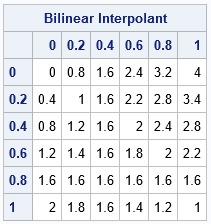The table shows the interpolant evaluated at the coordinates of a regular 6 x 6 grid. The column headers give the coordinate of the X variable and the row headers give the coordinates of the Y variable. You can see that each column and each row is an arithmetic sequence, which shows that the interpolant is linear on vertical and horizontal lines.

You can use a finer grid and a heat map to visualize the interpolant as a surface. Notice that in the previous table, the Y values increase as you go down the rows. If you want the Y axis to point up instead of down, you can reverse the rows of the grid (and the labels) before you create a heat map:

```/* increase the grid resolution and visualize by using a heat map */ xGrd = do(0, 1, 0.1); yGrd = do(0, 1, 0.1); t = ExpandGrid(yGrd, xGrd); /* want X changing fastest; put in 2nd col */ t = t[ ,{2 1}]; /* reverse the columns from (y,x) to (x,y) */ F = bilinearInterpSquare(z, t); Q = shape(F, ncol(yGrd), ncol(xGrd));   /* currently, the Y axis is pointing down. Flip it and the labels. */ H = Q[ nrow(Q):1, ]; reverseY = yGrd[ ,ncol(yGrd):1]; call heatmapcont(H) range={0 4} displayoutlines=0 xValues=xGrd yValues=reverseY colorramp=palette("Spectral", 7)[,7:1] title="Interpolation at Multiple Points in the Unit Square";```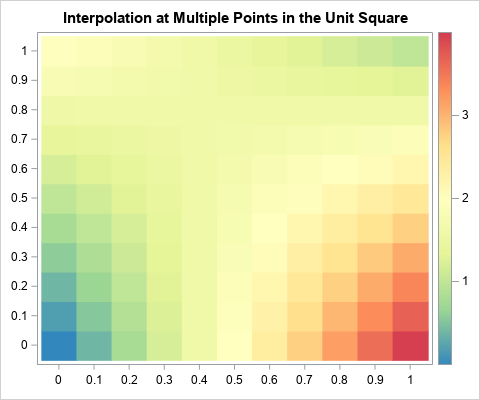The heat map agrees with the contour plot at the top of this article. The heat map also makes it easier to see that the bilinear interpolant is linear on each row and column.

In summary, you can create a short SAS/IML function that performs bilinear interpolation on the unit square. (You can download the SAS program that generates the tables and graphs in this article.) The interpolant is a quadratic saddle-shaped function inside the square. You can use the ideas in this article to create a function that performs bilinear interpolation on an arbitrary grid of fitting data. The next article shows a general function for bilinear interpolation in SAS.

The post What is bilinear interpolation? appeared first on The DO Loop.I've previously written about linear interpolation in one dimension. Bilinear interpolation is a method for two-dimensional interpolation on a rectangle. If the value of a function is known at the four corners of a rectangle, an interpolation scheme gives you a way to estimate the function at any point in the rectangle's interior. Bilinear interpolation is a weighted average of the values at the four corners of the rectangle. For an (x,y) position inside the rectangle, the weights are determined by the distance between the point and the corners. Corners that are closer to the point get more weight. The generic result is a saddle-shaped quadratic function, as shown in the contour plot to the right.

The Wikipedia article on bilinear interpolation provides a lot of formulas, but the article is needlessly complicated. The only important formula is how to interpolate on the unit square [0,1] x [0,1]. To interpolate on any other rectangle, simply map your rectangle onto the unit square and do the interpolation there. This trick works for bilinear interpolation because the weighted average depends only on the relative position of a point and the corners of the rectangle. Given a rectangle with lower-left corner (x0, y0) and upper right corner (x1, y1), you can map it into the unit square by using the transformation (x, y) → (u, v) where u = (x-x0)/(x1-x0) and v = (y-y0)/(y1-y0).

### Bilinear interpolation on the unit square

Suppose that you want to interpolate on the unit square. Assume you know the values of a continuous function at the corners of the square:

• z00 is the function value at (0,0), the lower left corner
• z10 is the function value at (1,0), the lower right corner
• z01 is the function value at (0,1), the upper left corner
• z11 is the function value at (1,1), the upper right corner

If (x,y) is any point inside the unit square, the interpolation at that point is the following weighted average of the values at the four corners:
F(x,y) = z00*(1-x)*(1-y) + z10*x*(1-y) + z01*(1-x)*y + z11*x*y

Notice that the interpolant is linear with respect to the values of the corners of the square. It is quadratic with respect to the location of the interpolation point. The interpolant is linear along every horizontal line (lines of constant y) and along every vertical line (lines of constant x).

### Implement bilinear interpolation on the unit square in SAS

Suppose that the function values at the corners of a unit square are z00 = 0, z10 = 4, z01 = 2, and z11 = 1. For these values, the bilinear interpolant on the unit square is shown at the top of this article. Notice that the value of the interpolant at the corners agrees with the data values. Notice also that the interpolant is linear along each edge of the square. It is not easy to see that the interpolant is linear along each horizontal and vertical line, but that is true.

In SAS, you can use the SAS/IML matrix language to define a function that performs bilinear interpolation on the unit square. The function takes two arguments:

• z is a four-element that specifies the values of the data at the corners of the square. The values are specified in the following order: lower left, lower right, upper left, and upper right. This is the "fitting data."
• t is a k x 2 matrix, where each row specifies a point at which to interpolate. This is the "scoring data."

In a matrix language such as SAS/IML, the function can be written in vectorized form without using any loops:

```proc iml; start bilinearInterpSquare(z, t); z00 = z; z10 = z; z01 = z; z11 = z; x = t[,1]; y = t[,2]; cx = 1-x; /* cx = complement of x */ return( (z00*cx + z10*x)#(1-y) + (z01*cx + z11*x)#y ); finish;   /* specify the fitting data */ z = {0 4, /* bottom: values at (0,0) and (1,0) */ 2 1}; /* top: values at (0,1) and (1,1) */ print z[c={'x=0' 'x=1'} r={'y=0' 'y=1'}];   t = {0.5 0, /* specify the scoring data */ 0 0.25, 1 0.66666666, 0.5 0.75, 0.75 0.5 }; F = bilinearInterpSquare(z, t); /* test the bilinear interpolation function */ print t[c={'x' 'y'} format=FRACT.] F;```For points on the edge of the square, you can check a few values in your head:

• The point (1/2, 0) is halfway between the corners with values 0 and 4. Therefore the interpolation gives 2.
• The point (0, 1/4) is a quarter of the way between the corners with values 0 and 2. Therefore the interpolation gives 0.5.
• The point (1, 2/3) is two thirds of the way between the corners with values 4 and 1. Therefore the interpolation gives 2.

The other points are in the interior of the square. The interpolant at those points is a weighted average of the corner values.

### Visualize the interpolant function

Let's use the bilinearInterpSquare function to evaluate a grid of points. You can use the ExpandGrid function in SAS/IML to generate a grid of points. When we look at the values of the interpolant on a grid or in a heat map, we want the X values to change for each column and the Y values to change for each row. This means that you should reverse the usual order of the arguments to the ExpandGrid function:

```/* for visualization, reverse the arguments to ExpandGrid and then swap 1st and 2nd columns of t */ xGrd = do(0, 1, 0.2); yGrd = do(0, 1, 0.2); t = ExpandGrid(yGrd, xGrd); /* want X changing fastest; put in 2nd col */ t = t[ ,{2 1}]; /* reverse the columns from (y,x) to (x,y) */ F = bilinearInterpSquare(z, t); Q = shape(F, ncol(yGrd), ncol(xGrd)); print Q[r=(char(YGrd,3)) c=(char(xGrd,3)) label="Bilinear Interpolant"];```The table shows the interpolant evaluated at the coordinates of a regular 6 x 6 grid. The column headers give the coordinate of the X variable and the row headers give the coordinates of the Y variable. You can see that each column and each row is an arithmetic sequence, which shows that the interpolant is linear on vertical and horizontal lines.

You can use a finer grid and a heat map to visualize the interpolant as a surface. Notice that in the previous table, the Y values increase as you go down the rows. If you want the Y axis to point up instead of down, you can reverse the rows of the grid (and the labels) before you create a heat map:

```/* increase the grid resolution and visualize by using a heat map */ xGrd = do(0, 1, 0.1); yGrd = do(0, 1, 0.1); t = ExpandGrid(yGrd, xGrd); /* want X changing fastest; put in 2nd col */ t = t[ ,{2 1}]; /* reverse the columns from (y,x) to (x,y) */ F = bilinearInterpSquare(z, t); Q = shape(F, ncol(yGrd), ncol(xGrd));   /* currently, the Y axis is pointing down. Flip it and the labels. */ H = Q[ nrow(Q):1, ]; reverseY = yGrd[ ,ncol(yGrd):1]; call heatmapcont(H) range={0 4} displayoutlines=0 xValues=xGrd yValues=reverseY colorramp=palette("Spectral", 7)[,7:1] title="Interpolation at Multiple Points in the Unit Square";```The heat map agrees with the contour plot at the top of this article. The heat map also makes it easier to see that the bilinear interpolant is linear on each row and column.

In summary, you can create a short SAS/IML function that performs bilinear interpolation on the unit square. (You can download the SAS program that generates the tables and graphs in this article.) The interpolant is a quadratic saddle-shaped function inside the square. You can use the ideas in this article to create a function that performs bilinear interpolation on an arbitrary grid of fitting data. The next article shows a general function for bilinear interpolation in SAS.

The post What is bilinear interpolation? appeared first on The DO Loop.Dr. Ryan McGarry might have the most uncanny timing of any documentary producer in history. Just weeks before the novel coronavirus began to saturate headlines, the emergency medicine physician’s Netflix documentary series Pandemic hit the screens of millions of viewers. Six months ago, many of the subjects featured in the [...]